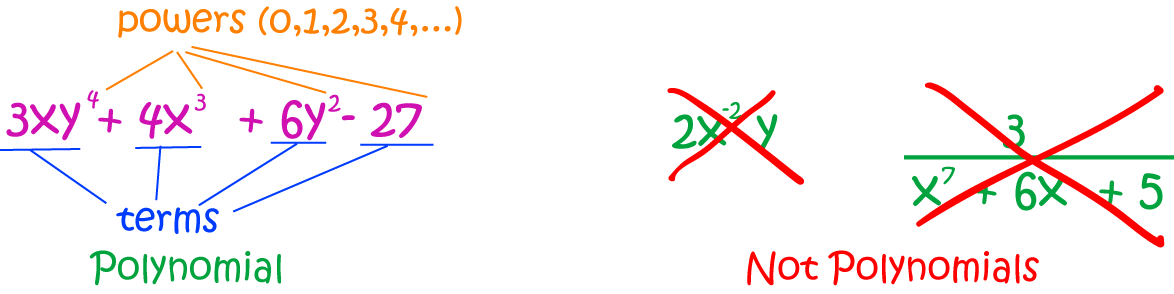# Definition of PolynomialA polynomial is a function that involves constants and positive powers of variables that are combined together using addition, subtraction and multiplication.

Polynomials do not include:

• Division by a variable
• Negative powers of variables
• An infinite number of terms
• Functions other than powers of $x$ and constants

### Description

The aim of this dictionary is to provide definitions to common mathematical terms. Students learn a new math skill every week at school, sometimes just before they start a new skill, if they want to look at what a specific term means, this is where this dictionary will become handy and a go-to guide for a student.

### Audience

Year 1 to Year 12 students

### Learning Objectives

Learn common math terms starting with letter P

Author: Subject Coach
You must be logged in as Student to ask a Question.